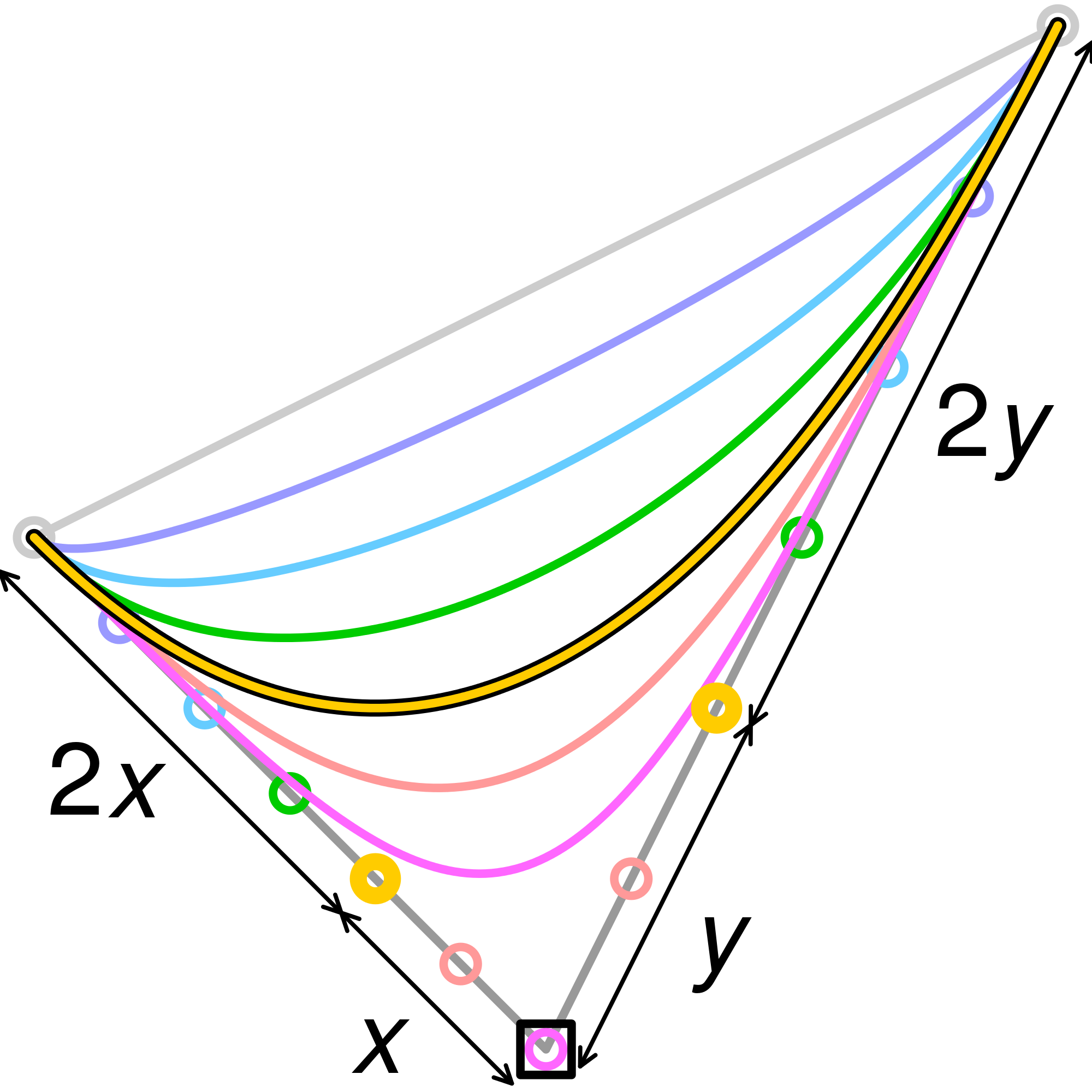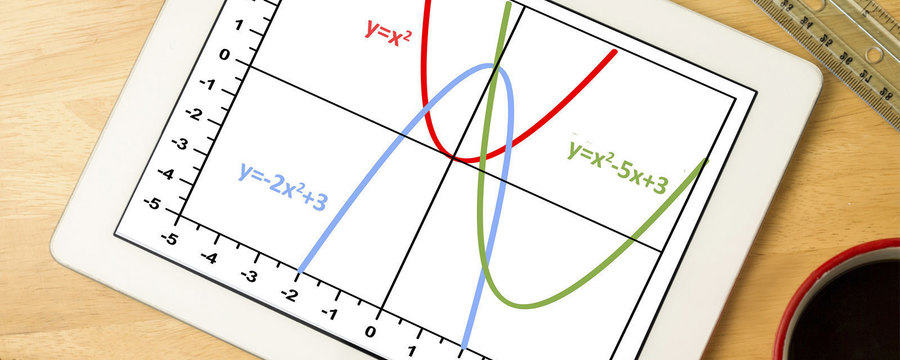﻿ De Casteljau Bezier curves and design
•# De Casteljau Bezier curves and design

A brief introduction to an important approach to curves, discovered by two French car engineers around 1960.Curves have been studied for thousands of years, so it was a major surprise when two French engineers, working for rival car companies, discovered around 1960 a lovely new way of thinking about curves. Their ideas proved especially useful for design work, and have since become a staple tool of designers around the world.
In this step, we will get an overview of how de Casteljau Bezier curves are used in practice for design.

## Two brilliant French car company engineers

Paul de Casteljau worked for Citroen, and Pierre Bezier worked for Renault. Around 1960, they independently discovered a new, and surprisingly simple way of specifying curves that has revolutionised modern design.
They noticed that we could specify curves like parabolas, and cubics, not just by equations, or points that they pass through, but by control points that direct the curve near its endpoints. Although the idea works for higher degree curves as well, it is especially simple and powerful for quadratic and cubic curves.
The key idea is to generalise the linear motion of a point moving between two endpoints $$\normalsize{A}$$ and $$\normalsize{B}$$ to a quadratic motion determined by three points $$\normalsize{A}$$, $$\normalsize{B}$$ and $$\normalsize{C}$$.
Suppose that $$\normalsize{P}$$ traverses the segment $$\normalsize{AB}$$ from $$\normalsize{t=0}$$ to $$\normalsize{t=1}$$, and let $$\normalsize{R}$$ be a similiar point which traverses the segment $$\normalsize{BC}$$ as $$\normalsize{t}$$ goes from $$\normalsize{0}$$ to $$\normalsize{1}$$.
We define the line $${\normalsize PR}$$ whose position depends on $$t$$. Now comes the tricky bit: consider yet another point $$\normalsize{O}$$ which traverses the moving segment $$\normalsize{PR}$$ also linearly as $$\normalsize{t}$$ goes from $$\normalsize{0}$$ to $$\normalsize{1}$$. Then the combined motions give $$\normalsize{O}$$ a movement along a parabolic curve!The points $$\normalsize{A}$$, $$\normalsize{B}$$ and $$\normalsize{C}$$ are called the control points of the curve. The control points $$\normalsize{A}$$ and $$\normalsize{C}$$ fix the end points of the curve, while $$\normalsize{B}$$ determines the tangent lines to the curve at these end points. It turns out that if we have a parabola through two fixed points, then its shape is completely determined by these two tangent directions.
So with the de Casteljau Bezier framework, just three points determine a nice little quadratic arc.

Consider the arc of the de Casteljau Bezier curve (the parabola below in green) with control points $$\normalsize{A=[1, 1]}$$ $$\normalsize{B=[3,4]}$$ and $$\normalsize{C=[7, 1]}$$.Then we know from our discussion of parametric lines that
$\Large{P(t)=(1-t)[1, 1]+t[3,4]=[1+2t,1+3t]}$
and
$\Large{R(t)=(1-t)[3,4]+t[7,1]=[3+4t,4-3t]}.$
So
$\Large{O(t)=(1-t)P+tR=(1-t)[1+2t,1+3t]+t[3+4t,4-3t]}$
which becomes, after some algebra that you can check,
$\Large{O(t)=[2t^2+4t+1,-6t^2+6t+1]}.$
This is a parametric form for the parabola!

## The general form

As we have mentioned before, sometimes things become a bit clearer if we make them more general! Let’s look at the same computation we just did, but with general points. The point $$\normalsize P$$ on the line $$\normalsize AB$$ is determined by the parametric equation
${ \Large P(t) = (1-t) A+ tB }$
while the point $$\normalsize R$$ on the line $$\normalsize BC$$ is
${ \Large R(t) = (1-t)B + t C }.$
But in addition the point $$\normalsize O$$ on $$\normalsize PR$$ is
${ \Large O(t) = (1-t)P + t R=(1-t)\left((1-t)A+tB\right)+t\left((1-t)B+tC\right)}.$
So we get that the parametric form of the quadratic de Casteljau Bezier curve with control points $$\normalsize A,B,C$$ is
${ \Large O(t) = (1-t)^2 A+ 2t(1-t)B + t^2 C }.$
Q1 (E): What is the sum of the coefficients $$\normalsize (1-t)^2, 2t(1-t), t^2$$? These special coefficients are also known as Bernstein basis polynomials.
Q2 (M): What is the de Casteljau Bezier curve with control points $$\normalsize P_0 = [0,-1], P_1 = [1,0], P_2 = [0,1]$$?

## Cubic de Casteljau Bezier curves

Let’s mention that this process of building up more complicated curves can be used to go from quadratic to cubic de Casteljau Bezier curves. These now have four control points. Can you figure out what is going on in the following figure?In summary, de Casteljau Bezier curves allow us to efficiently record and change information about the shapes of curves without having to rely on pictorial or Cartesian representations. That is why these curves are used extensively in design programs, such as Illustrator, CorelDraw, AutoCad, etc., and why they are the mathematical basis for major font families, such as True Type fonts. Now you can go design a car. Have fun!

## Discussion

The curves of de Casteljau and Bezier are often called just Bezier curves, which is a bit unfortunate, as de Casteljau deserves half the credit. You may very well have used tools to generate such curves in graphics or Cad programs. Please tell us about your experience with these curves if you have something to share.

A1. The coefficients sum to $$\normalsize 1$$.
A2. The curve is $$\normalsize p(t) = [2t(1-t),2t-1]$$.

#### Maths for Humans: Linear, Quadratic & Inverse Relations